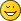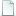Points on a circle primitive

Points on a circle primitive
#1
Hi all,

Below is a perl script to take a bunch of angles and convert them to points on the edge of a circle primitve (ie. a 16 or 48 point polygon). The comments up top describe how to use it. It's designed to make generating rims on the bottom of curved parts relatively easy when there's only a few segments required and hand-coding makes more sense than using Philo's wonderful toys.

Tim

Code:
#! /usr/bin/perl
# ang2point.pl [-r RAD] [-t THICK] [-s SEGS] ang1 ang2 ... angn
#
# THICK defaults to none
# SEGS defaults to 16
#
# with SEGS segments. If THICK is specified it also shows the
#
# It scales the radius at each angle to ensure it lies on the
# SEG-sided polygon that approximates the circle.
#

use POSIX;

\$segs=16;
@angs=();
\$thick=0;

for (\$ia=0; \$ia<=\$#ARGV; \$ia++) {
\$opt=lc(\$ARGV[\$ia]);
if (\$opt eq '-r') {
\$ia++;
} elsif (\$opt eq '-t') {
\$ia++;
\$thick=\$ARGV[\$ia];
} elsif (\$opt eq '-s') {
\$ia++;
\$segs=\$ARGV[\$ia];
} else {
push @angs, \$opt;
}
}

\$nang=\$#angs+1;
print "0 // ang2point.pl ".join(' ', @ARGV)."\n";
print "0 // Calculating \$nang points at outer radius \$rad on \$segs segments\n";
if (\$thick>0) {
}

@reffs=();
@rieffs=();

\$kk=3.141592635/180;
foreach \$ang (@angs) {
\$f=\$ang/360;
\$a0=floor(\$f*\$segs)/\$segs*360;
\$ar=\$ang-\$a0;
#print "\$a0 + \$ar\n";

\$c0=cos(\$kk*\$a0);
\$s0=sin(\$kk*\$a0);
\$cf=cos(\$kk*(\$a0+360/\$segs));
\$sf=sin(\$kk*(\$a0+360/\$segs));

\$ct=cos(\$kk*\$ang);
\$st=sin(\$kk*\$ang);

\$scale=(\$c0*\$sf-\$s0*\$cf)/((\$sf-\$s0)*\$ct-(\$cf-\$c0)*\$st);
push @reffs, \$reff;
push @rieffs, \$rieff;
}

@pts=();
@ipts=();
foreach \$ia (0..\$#angs) {
\$x=\$reffs[\$ia]*cos(\$kk*\$angs[\$ia]);
\$z=\$reffs[\$ia]*sin(\$kk*\$angs[\$ia]);
push @pts, sprintf("0 // Point %2d: %.3f 0 %.3f\n",
\$ia+1, \$x, \$z);
if (\$thick>0) {
\$x=\$rieffs[\$ia]*cos(\$kk*\$angs[\$ia]);
\$z=\$rieffs[\$ia]*sin(\$kk*\$angs[\$ia]);
push @ipts, sprintf("0 // Inner point %2d: %.3f 0 %.3f\n",
\$ia+1, \$x, \$z);
}
}

print @pts;
print @ipts;
Re: Points on a circle primitive
#2
Looks interesting - I agree that my "wonderful" tools can be clumsy to useWould you have a sample run (not so familar with perl...)
Re: Points on a circle primitive
#3
Not so much clumsy in this case as not quite the right tool for the job. That said I'll be using ytruder for certainI plan to demonstrate it properly when I get a chance to redo the base of 65188. I'll try to remember to snap the stages. But...

Code:
0 // ang2point.pl -r 120 -t 4 -s 48 0 7.5 11
0 // Calculating 3 points at outer radius 120 on 48 segments
0 // Also calculating inner radius 116
0 // Point  1: 120.000 0 0.000
0 // Point  2: 118.973 0 15.663
0 // Point  3: 117.544 0 22.848
0 // Inner point  1: 116.000 0 0.000
0 // Inner point  2: 115.008 0 15.141
0 // Inner point  3: 113.626 0 22.087

Tim

PS. This one should be very easy to convert to C. But processing arguments is simpler in perl so I chose that option.
Re: Points on a circle primitive
#4

Attached is the stages I took. Finally I ran ytruder on itAttached FilesRimWithWorking2.dat (Size: 1.12 KB / Downloads: 0)
« Next Oldest | Next Newest »

Forum Jump:

Users browsing this thread: 1 Guest(s)